# polyNormal

Below is a demonstration of the features of the polyNormal function

## Contents

```clear; close all; clc;
```

## Syntax

[varargout]=polyNormal(V_poly);

UNDOCUMENTED

## Examples

Plot settings

```markerSize=50;
lineWidth=2;
fontSize=25;
```

## Example 1: Getting point-wise normal vectors for a 2D polygon

```x=linspace(0,2*pi,15)';
y=sin(x);
V=[x y];
```

Using all defaults:

```N=polyNormal(V)
```
```N =

-0.6951    0.7190
-0.6568    0.7541
-0.5162    0.8564
-0.2103    0.9776
0.2103    0.9776
0.5162    0.8564
0.6568    0.7541
0.6951    0.7190
0.6568    0.7541
0.5162    0.8564
0.2103    0.9776
-0.2103    0.9776
-0.5162    0.8564
-0.6568    0.7541
-0.6951    0.7190

```

## Example 2: Getting point-wise normal vectors for a 3D polygon

```t=linspace(0,2*pi,15)';
x=cos(t);
y=sin(t);
z=2*(t./(2*pi));
V=[x y z];
```

Using all defaults:

```N=polyNormal(V)
```
```N =

-0.9749   -0.2225         0
-0.9010   -0.4339         0
-0.6235   -0.7818         0
-0.2225   -0.9749         0
0.2225   -0.9749         0
0.6235   -0.7818         0
0.9010   -0.4339         0
1.0000   -0.0000         0
0.9010    0.4339         0
0.6235    0.7818         0
0.2225    0.9749         0
-0.2225    0.9749         0
-0.6235    0.7818         0
-0.9010    0.4339         0
-0.9749    0.2225         0

```

## Example 3: Getting point-wise normal vectors for a non-closed polygon

Specifying options

```optionStruct.closeLoopOpt=false; %Wether the input should be considered a closed curve
optionStruct.type='vertex'; % 'vertex'/'node' or 'segment'/'edge'
optionStruct.zDir=[0 1 1]; %z-direction

[N,VN]=polyNormal(V,optionStruct);
```
```cFigure;  hold on;
hp1=plotV(V,'k.-','MarkerSize',markerSize,'LineWidth',lineWidth);
hp2=quiverVec(VN,N,0.5,'r');
legend([hp1 hp2],{'Polygon','vertex normal vectors'});
axisGeom(gca,fontSize);
drawnow;
```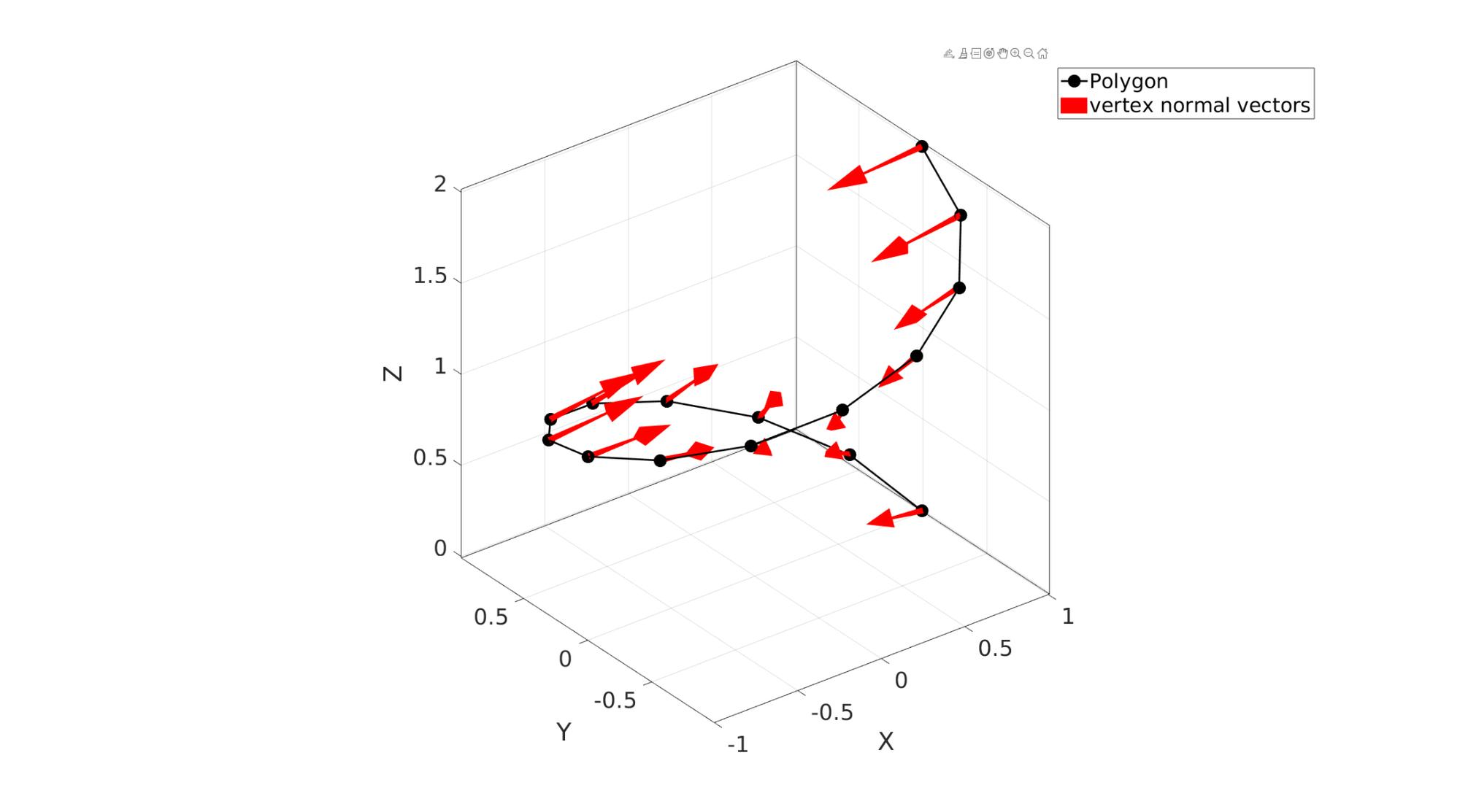## Example 4: Getting segment-wise normal vectors for a non-closed polygon

```optionStruct.closeLoopOpt=false; %Wether the input should be considered a closed curve
optionStruct.type='segment'; % 'vertex'/'node' or 'segment'/'edge'
optionStruct.zDir=[0 0 1]; %z-direction

[N,VN]=polyNormal(V,optionStruct);
```
```cFigure;  hold on;
hp1=plotV(V,'k.-','MarkerSize',markerSize,'LineWidth',lineWidth);
hp2=quiverVec(VN,N,0.5,'b');
legend([hp1 hp2],{'Polygon','segment normal vectors'});
axis equal; grid on; box on;
set(gca,'FontSize',fontSize);
drawnow;
```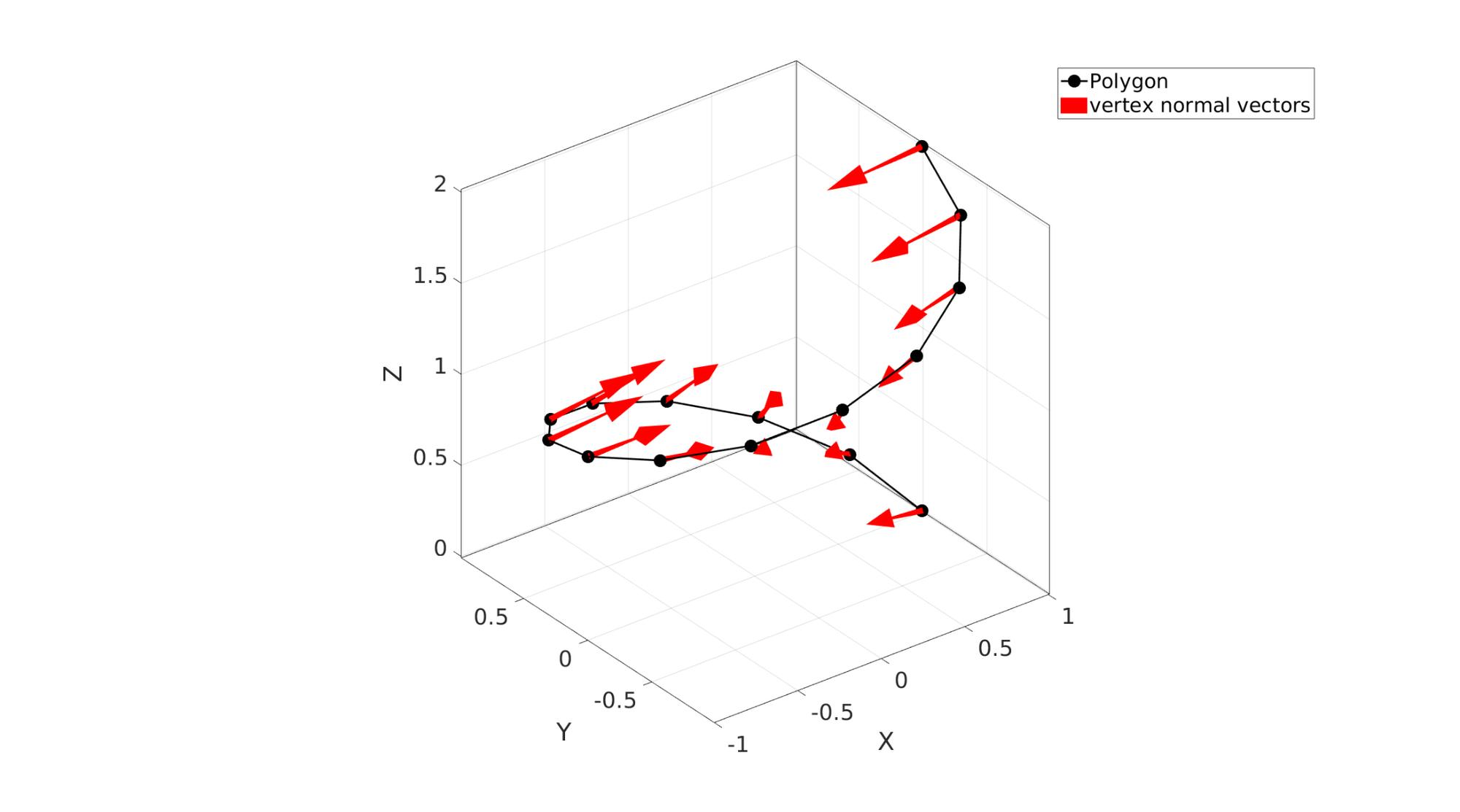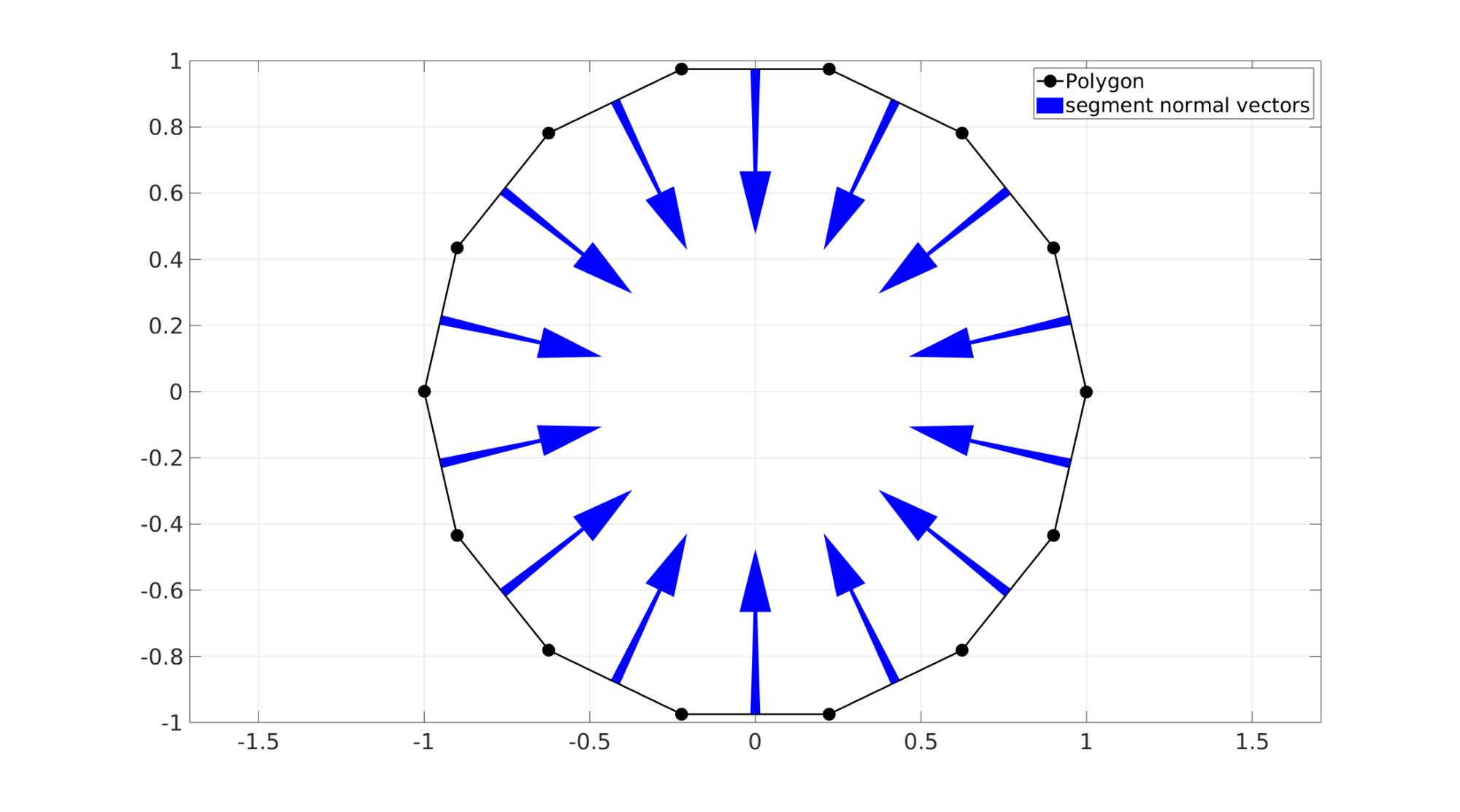## Example 5: Getting point-wise normal vectors for a closed polygon

```t=linspace(0,2*pi,25)'; t=t(1:end-1);
r=3;
x=r*cos(t);
y=r*sin(t);
V=[x y];

optionStruct.closeLoopOpt=true; %Wether the input should be considered a closed curve
optionStruct.type='vertex'; % 'vertex'/'node' or 'segment'/'edge'
optionStruct.zDir=[0 0 1]; %z-direction

N=polyNormal(V,optionStruct);
```
```cFigure;  hold on;
hp1=plotV(V,'k.-','MarkerSize',markerSize,'LineWidth',lineWidth);
hp2=quiverVec(V,N,1,'r');
legend([hp1 hp2],{'Polygon','segment normal vectors'});
axis equal; grid on; box on;
set(gca,'FontSize',fontSize);
drawnow;
```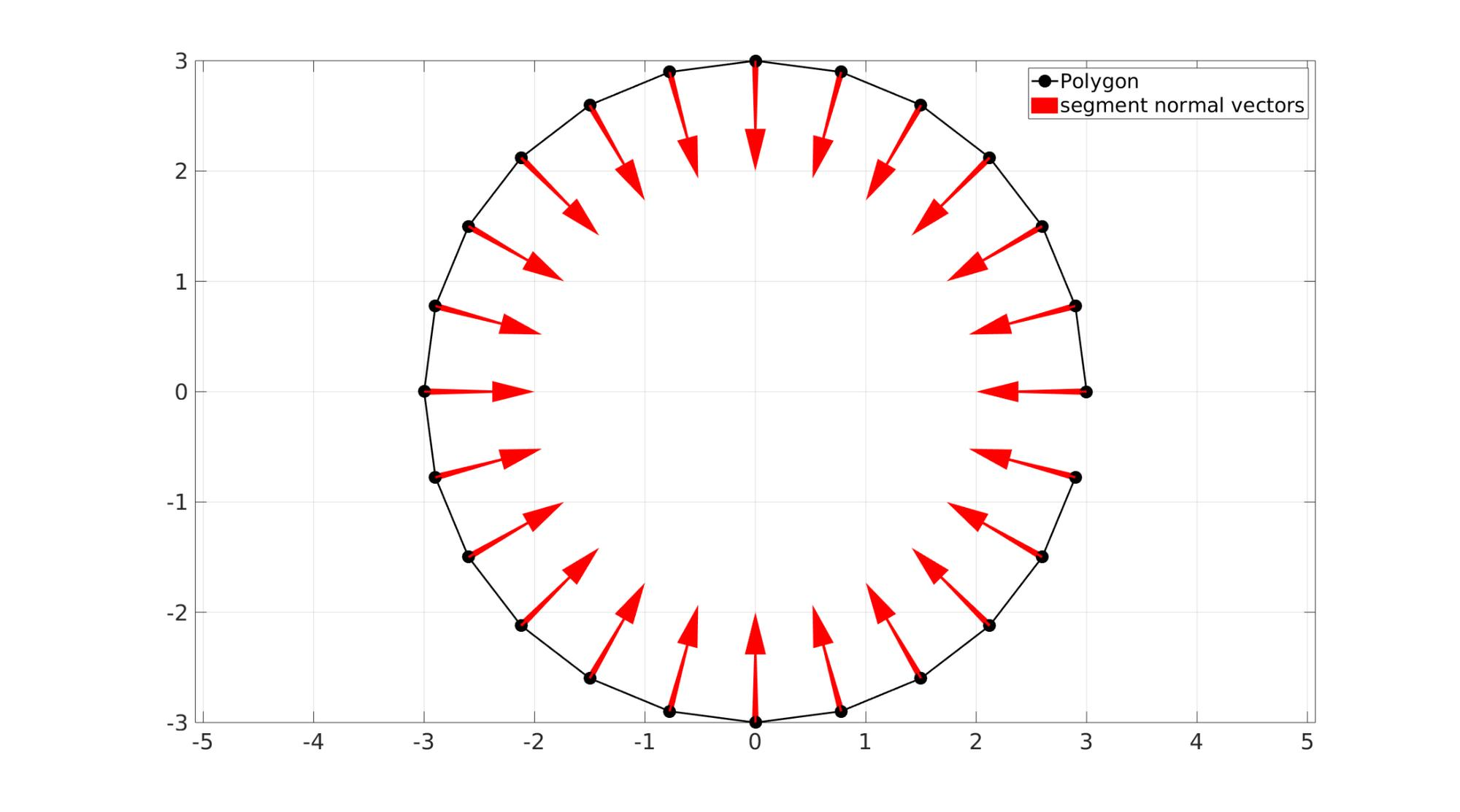## Example 6: Getting segment-wise normal vectors for a closed polygon

```optionStruct.closeLoopOpt=true; %Wether the input should be considered a closed curve
optionStruct.type='segment'; % 'vertex'/'node' or 'segment'/'edge'
optionStruct.zDir=[0 0 1]; %z-direction

[N,VN]=polyNormal(V,optionStruct);
```
```cFigure;  hold on;
hp1=plotV(V,'k.-','MarkerSize',markerSize,'LineWidth',lineWidth);
hp2=quiverVec(VN,N,1,'b');
legend([hp1 hp2],{'Polygon','segment normal vectors'});
axis equal; grid on; box on;
set(gca,'FontSize',fontSize);
drawnow;
```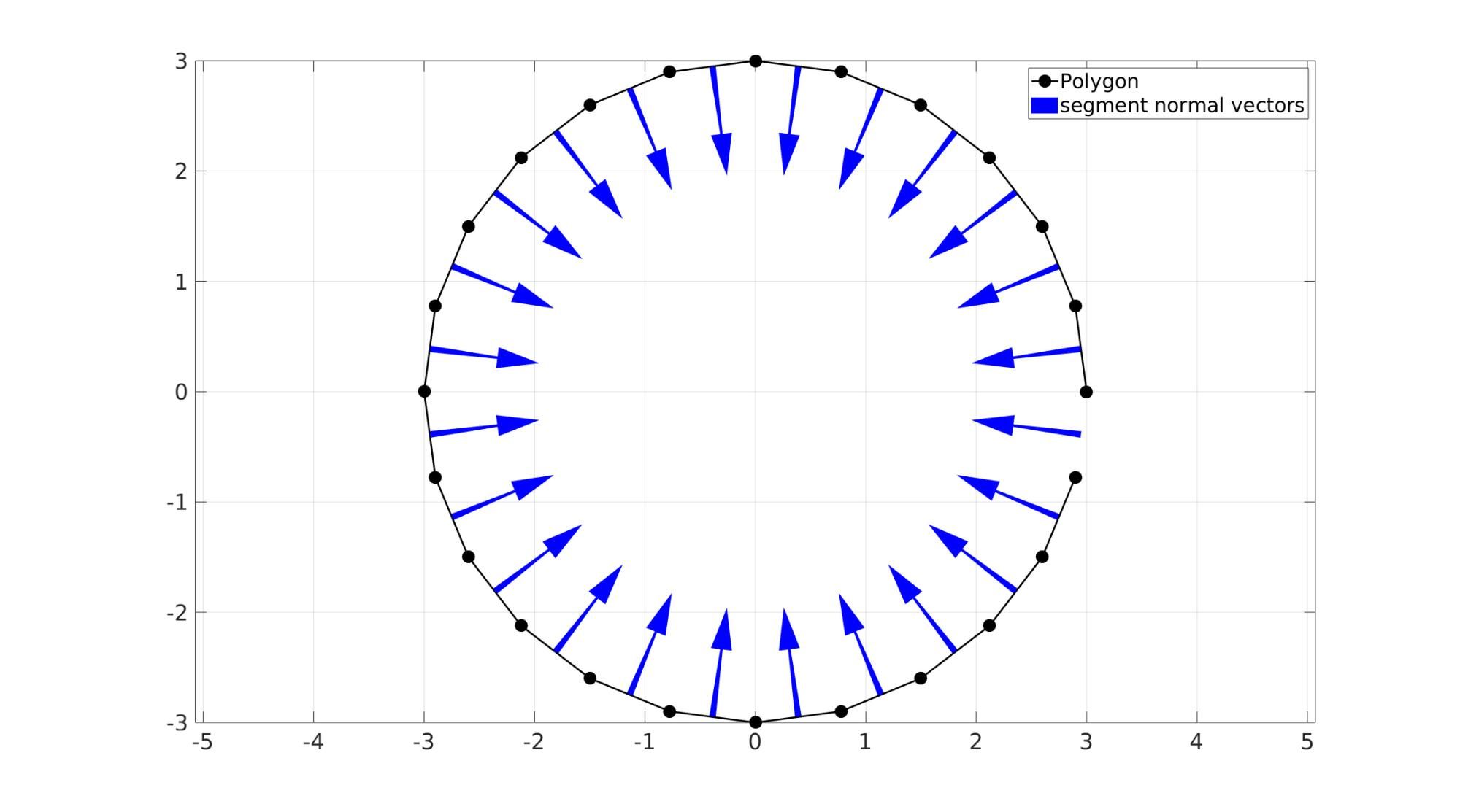GIBBON www.gibboncode.org

Kevin Mattheus Moerman, [email protected]

GIBBON footer text

GIBBON: The Geometry and Image-based Bioengineering add-On. A toolbox for image segmentation, image-based modeling, meshing, and finite element analysis.

Copyright (C) 2006-2023 Kevin Mattheus Moerman and the GIBBON contributors

This program is free software: you can redistribute it and/or modify it under the terms of the GNU General Public License as published by the Free Software Foundation, either version 3 of the License, or (at your option) any later version.

This program is distributed in the hope that it will be useful, but WITHOUT ANY WARRANTY; without even the implied warranty of MERCHANTABILITY or FITNESS FOR A PARTICULAR PURPOSE. See the GNU General Public License for more details.

You should have received a copy of the GNU General Public License along with this program. If not, see http://www.gnu.org/licenses/.